$$\require{cancel}$$

# 8.9: Absorption and Stimulated Emission of Radiation

Let us use some of the results of time-dependent perturbation theory to investigate the interaction of an atomic electron with classical (i.e., non-quantized) electromagnetic radiation.

The unperturbed Hamiltonian is

$$H_0 = \frac{p^2}{2 \,m_e} + V_0(r).$$ \ref{862}

The standard classical prescription for obtaining the Hamiltonian of a particle of charge $$q$$ in the presence of an electromagnetic field is

$${\bf p}$$ $$\rightarrow {\bf p} - q\,{ \bf A},$$ \ref{863} $$H$$ $$\rightarrow H-q\,\phi,$$ \ref{864}

where $${\bf A}(\bf r)$$ is the vector potential and $$\phi({\bf r})$$ is the scalar potential. Note that

$${\bf E}$$ $$= - \nabla\phi - \frac{\partial {\bf A}}{\partial t},$$ \ref{865} $${\bf B}$$ $$= \nabla\times {\bf A}.$$ \ref{866}

This prescription also works in quantum mechanics. Thus, the Hamiltonian of an atomic electron placed in an electromagnetic field is

$$H = \frac{\left\vert{\bf p} + e\, {\bf A}\right\vert^{\,2} }{2\,m_e}- e \,\phi + V_0(r),$$ \ref{867}

where $${\bf A}$$ and $$\phi$$ are real functions of the position operators. The above equation can be written

$$H = \frac{ \left(p^2 +e \,{\bf A}\cdot {\bf p} +e \,{\bf p}\cdot{\bf A} + e^2 A^2\right)}{2\,m_e}- e \,\phi + V_0(r).$$ \ref{868}

Now,

$${\bf p}\cdot {\bf A} = {\bf A}\cdot {\bf p},$$ \ref{869}

provided that we adopt the gauge $$\nabla\cdot{\bf A} = 0$$ . Hence,

$$H = \frac{p^2}{2\,m_e} -\frac{e\,{\bf A}\cdot{\bf p}}{m_e} +\frac{ e^2 A^2}{2\,m_e}- e\, \phi + V_0(r).$$ \ref{870}

Suppose that the perturbation corresponds to a monochromatic plane-wave, for which

$$\phi$$ $$= 0,$$ \ref{871} $${\bf A}$$ $$= 2\, A_0 \,$$$$\mbox{\boldmath$$$$\,\cos\left (\frac{\omega}{c} \,{\bf n}\cdot{\bf x} - \omega\, t\right),$$ \ref{872}

where $$\epsilon$$ and $${\bf n}$$ are unit vectors that specify the direction of polarization and the direction of propagation, respectively. Note that $$\epsilon$$ $$\cdot{\bf n} = 0$$ . The Hamiltonian becomes

$$H = H_0 + H_1(t),$$ \ref{873}

with

$$H_0 = \frac{p^2}{2\,m_e} + V(r),$$ \ref{874}

and

$$H_1 \simeq \frac{e\,{\bf A}\cdot{\bf p}}{m_e},$$ \ref{875}

where the $$A^2$$ term, which is second order in $$A_0$$ , has been neglected.

The perturbing Hamiltonian can be written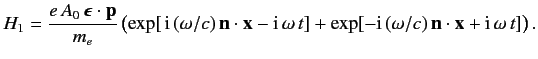\ref{876}

This has the same form as Equation \ref{850}, provided that

$$V = - \frac{e \,A_0\, \mbox{\boldmath$$ \ref{877}

It is clear, by analogy with the previous analysis, that the first term on the right-hand side of Equation \ref{876} describes the absorption of a photon of energy $$\hbar\,\omega$$ , whereas the second term describes the stimulated emission of a photon of energy $$\hbar\,\omega$$ . It follows from Equations \ref{859} and \ref{860} that the rates of absorption and stimulated emission are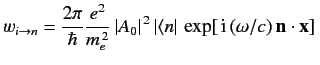$$\mbox{\boldmath$$$$\cdot{\bf p} \,\vert i\rangle\vert^{\,2}\, \delta(E_n-E_i -\hbar\,\omega),$$ \ref{878}

and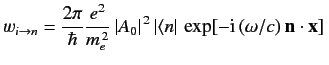$$\mbox{\boldmath$$$$\cdot{\bf p} \,\vert i\rangle\vert^{\,2}\, \delta(E_n-E_i +\hbar\,\omega),$$ \ref{879}

respectively.

Now, the energy density of a radiation field is

$$U = \frac{1}{2}\left(\frac{\epsilon_0\,E_0^{\,2}}{2}+ \frac{B_0^{\,2}}{2\,\mu_0} \right),$$ \ref{880}

where $$E_0$$ and $$B_0=E_0/c= 2\,A_0\,\omega/c$$ are the peak electric and magnetic field-strengths, respectively. Hence,

$$U = 2\,\epsilon_0\,c\,\omega^2\,\vert A_0\vert^{\,2},$$ \ref{881}

and expressions \ref{878} and \ref{879} become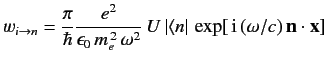$$\mbox{\boldmath$$$$\cdot{\bf p} \,\vert i\rangle\vert^{\,2}\, \delta(E_n-E_i -\hbar\,\omega),$$ \ref{882}

and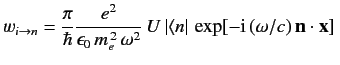$$\mbox{\boldmath$$$$\cdot{\bf p} \,\vert i\rangle\vert^{\,2}\, \delta(E_n-E_i +\hbar\,\omega),$$ \ref{883}

respectively. Finally, if we imagine that the incident radiation has a range of different frequencies, so that

$$U = \int d\omega\,u(\omega),$$ \ref{884}

where $$d\omega\,u(\omega)$$ is the energy density of radiation whose frequency lies in the range $$\omega$$ to $$\omega+d\omega$$ , then we can integrate our transition rates over $$\omega$$ to give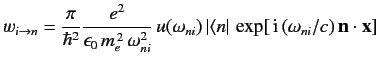$$\mbox{\boldmath$$$$\cdot{\bf p} \,\vert i\rangle\vert^{\,2}$$ \ref{885}

for absorption, and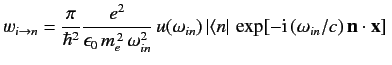$$\mbox{\boldmath$$$$\cdot{\bf p} \,\vert i\rangle\vert^{\,2}$$ \ref{886}

for stimulated emission. Here, $$\omega_{ni} = (E_n-E_i)/\hbar>0$$ and $$\omega_{in} = (E_i-E_n)/\hbar>0$$ . Furthermore, we are assuming that the radiation is incoherent, so that intensities can be added.

## Contributors

• Richard Fitzpatrick (Professor of Physics, The University of Texas at Austin)

$$\newcommand {\ltapp} {\stackrel {_{\normalsize<}}{_{\normalsize \sim}}}$$ $$\newcommand {\gtapp} {\stackrel {_{\normalsize>}}{_{\normalsize \sim}}}$$ $$\newcommand {\btau}{\mbox{\boldmath\tau}}$$ $$\newcommand {\bmu}{\mbox{\boldmath\mu}}$$ $$\newcommand {\bsigma}{\mbox{\boldmath\sigma}}$$ $$\newcommand {\bOmega}{\mbox{\boldmath\Omega}}$$ $$\newcommand {\bomega}{\mbox{\boldmath\omega}}$$ $$\newcommand {\bepsilon}{\mbox{\boldmath\epsilon}}$$